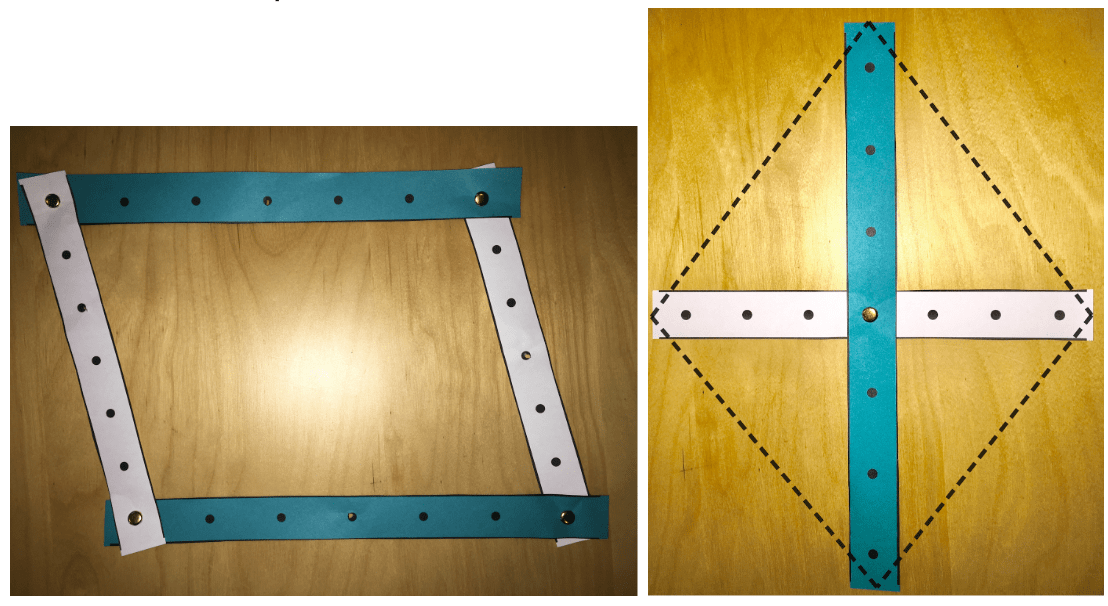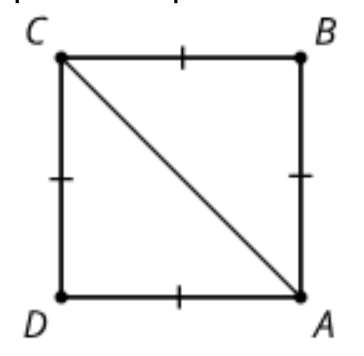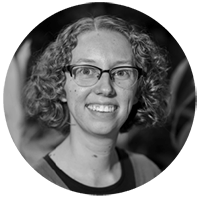Supporting high-school students to write detailed, precise proofs is challenging. Learn about some of the design elements that IM used to invite students to a deep exploration of proof.

In IM’s high school Geometry curriculum, students are asked to engage in the proof cycle. The cycle includes: experiment, conjecture, convince themselves, convince others, read proofs, critique proofs, and write proofs. Students don’t complete the entire cycle for every activity, but they do engage in each step repeatedly throughout the course. Here are some of the principles that underlie our approach to proofs.

1. We begin with experimentation and conjecture.

For example, before students prove results about quadrilaterals, they experiment with hands-on or digital manipulatives that let them change the properties of the sides or diagonals and see what kinds of quadrilaterals result.Students generate many conjectures based on their experiments. Some examples might include:

• All rectangles are parallelograms.
• If a parallelogram has (at least) one right angle, then it is a rectangle.
• If a quadrilateral has two pairs of opposite sides that are congruent, then it is a parallelogram.
• If the diagonals of a quadrilateral both bisect each other, then the quadrilateral is a parallelogram.
• If the diagonals of a quadrilateral both bisect each other and are perpendicular, then the quadrilateral is a rhombus.

2. We ask students to convince themselves, then convince a partner.

In the next activity, we provide the above list of conjectures and ask students to work on their own first to complete the following steps:

1. Pick one conjecture and use the strips to convince yourself it is true.
1. Rewrite the conjecture to identify the given information and the statement to prove.
1. Draw a diagram of the situation. Mark the given information and any information you can figure out for sure.
1. Write a rough draft of how you might prove your conjecture is true.

Then, we ask students to work with a partner to read the rough draft of their proof. If it is convincing, students write a summary of their partner’s proof that shows why it works and why it will be useful. If it is not convincing, students suggest changes that will make the proof convincing.

Slowing down the proof cycle and separating out conjecturing, convincing yourself, making a clear conjecture with a diagram, drafting a proof, and then convincing someone else is important. This slower process helps students internalize that the goal of a proof is to convince someone that given a certain set of facts or assumptions, their conjecture must be true. We’ll say more in point 6 about why the summarizing step is important too.

3. We focus on proofs that will be useful.
Earlier in the unit, students proved the ASA, SAS, and SSS triangle congruence theorems. These proofs were long and filled with transformation statements and justifications. But once students write a proof, the conjecture becomes a theorem that encapsulates all of the transformations that are required to guarantee congruence.

Now, as they work with quadrilaterals, students could continue to write transformation proofs, defining certain transformations that would definitely work to take a certain side or angle onto another side or angle. But they have the alternative option of using a triangle congruence shortcut to summarize most of the argument. For example, we ask students to prove that all equilateral quadrilaterals are parallelograms using a diagram like this:Students could think about proving that a specific transformation that takes lines to parallel lines (such as a rotation by 180º around the midpoint of segment AC) will take line segment BC onto line segment AD. But because of all their work with triangle congruence shortcuts, they can also reason “because triangles ABC and ADC have all three pairs of corresponding sides congruent, there must be a transformation that takes one triangle onto the other (by SSS) and that transformation will take angle BCA onto angle DAC, so those angles must be congruent, which helps show that line segment BC is parallel to line segment AD.” The conjectures students are proving in the quadrilaterals activity will again be useful later in the course. But how did students get to the point where they could prove SSS, ASA, and SAS early in the course?

4. Students read and analyze proofs.
Students’ first introduction to the triangle congruence proofs was to read a proof and identify the lines in which the given information was used. Later, they read proofs with an error and are tasked with locating the incorrect statement. In the quadrilateral proofs lesson, students move from pairs to groups of 4, and decide if the rough draft proofs are convincing. If not, they suggest changes to make the argument stronger. These activities help students engage with the logic and format of proofs before they can produce a full proof on their own, and help build up the skills for reading and critiquing proofs that are part of being a community that engages in rigorous convincing.

5. Proof language comes from students’ ideas and gets formalized for easy repetition.
Students sometimes read a dialogue between two characters working on constructing an argument and help them complete their proof. We often ask students to write a convincing argument for something they’ve noticed or conjectured. During the synthesis of an activity in which we expect productive struggle (sometimes with emphasis on the struggle), we support teachers and students to sum up helpful phrases they used in proofs, and summarize what a successful proof must contain. We suggest that students refer back to previous sample proofs or their own proofs as they write new ones. Teachers create a display of helpful phrases that students can refer to. In addition, all of the assertions, definitions, and theorems that students might use in a proof are recorded in a reference chart. All of these supports mean that students are freed up to focus on the logic, not on the language, of proof.

6. Sometimes we focus on detailed proofs; often we focus on summaries.
We use activities such as “summarize this character’s proof in a sentence or two,” or “work with your partner to make a plan for the proof” that focus on the overall logical argument. Sometimes proofs have to have seemingly silly steps like “segment BC is congruent to segment BC,” and it’s easy to get bogged down in those details. We balance this with a focus on the big picture: “I can show that all three pairs of corresponding sides are congruent, so the triangles must be congruent (by SSS).” In the quadrilaterals lesson, the summarizing step happens twice. First, students write rough drafts with a partner before writing a detailed proof in a group. Then, at the end of class, students record on an exit ticket why it would be useful to know that all rectangles are parallelograms when proving that a quadrilateral with four right angles must have congruent opposite sides. This refocuses their attention on the main idea once more.

In this one lesson about quadrilaterals students completed nearly the entire proof cycle. They begin by experimenting and writing a conjecture. Then they choose a conjecture to prove. Proving is a multi-step process where students write a clear statement, create a matching diagram, and record a rough draft of their argument. Next, they work in groups to make sure the argument is convincing before writing a detailed argument. At the close of class students summarize why their new theorem is useful. While it is not possible to complete all these steps in every lesson, there are opportunities to complete each step throughout the year.

### Next Step

In your own Geometry course (or any other course!) this year, are there opportunities to give students a chance to conjecture and experiment before they prove? What might that look like?

• Are the proofs students write useful? Do students prove things that they will use later to prove other things?
• What opportunities are there to give students proofs to read, analyze, complete, or critique before they have to produce their own proofs?
• How can you help students focus on the overall logic of the proof as well as the details? Are there opportunities for students to focus on summaries or plans for writing a proof, in addition to writing their own complete proofs?
• How can the language of proof be templatized and formalized after students have had a chance to read examples and then generate their own language?
• The full IM Algebra 1, Geometry, and Algebra 2 Math curriculum is coming July 2019. Click here to be notified of upcoming announcements and updates.##### Max Ray-Riek

Max Ray-Riek is the Director of 6-12 Professional Development at Illustrative Mathematics and worked as a writer on the high school curriculum. Before coming to Illustrative Mathematics, Max worked for The Math Forum, focusing on fostering problem solving and making student thinking the center of math class. He is the lead author of Powerful Problem Solving: Activities for Sense Making with the Math Practices. Max is a former secondary mathematics teacher (and before that, preschool teacher) who finds the art and discipline of valuing each student’s ideas, and supporting all students to see themselves as people who can listen, connect, and act mathematically, to be the hardest, most rewarding, and most important work in the world. Max lives in Philadelphia with his wife, with whom he fosters puppies who will grow up to be trained to be service dogs.